# 带你读《计算机程序的构造和解释（原书第2版）典藏版》之一：构造过程抽象

+关注继续查看

Structure and Interpretation of Computer Programs，Second Edition[美] 杰拉尔德杰伊萨斯曼（Gerald Jay Sussman） 著

# 第1章 构造过程抽象

John Locke, An Essay Concerning Human Vnderstanding
（有关人类理解的随笔，1690）

Lisp并不是一个刻意的设计努力的结果，它以一种试验性的非正式的方式不断演化，以满足用户的需要和实际实现的各种考虑。Lisp的这种非官方演化持续了许多年，Lisp用户社团具有抵制制定这一语言的“官方”定义企图的传统。这种演化方式以及语言初始概念的灵活和优美，使得Lisp成为今天还在广泛使用的历史第二悠久的语言（只有Fortran比它更老）。这一语言还在不断调整，以便去包容有关程序设计的最新思想。正因为这样，今天的Lisp已经形成了一族方言，它们共享着初始语言的大部分特征，也可能有这样或那样的重要差异。用于本书的Lisp方言名为Scheme2。

## 1.1 程序设计的基本元素

• 基本表达形式，用于表示语言所关心的最简单的个体。
• 组合的方法，通过它们可以从较简单的东西出发构造出复合的元素。
• 抽象的方法，通过它们可以为复合对象命名，并将它们当作单元去操作。

### 1.1.1 表达式

486

486

(+ 137 349)
486
(- 1000 334)
666
(* 5 99)
495
(/ 10 5)
2
(+ 2.7 10)
12.7

(+ 21 35 12 7)
75
(* 25 4 12)
1200

(+ (* 3 5) (- 10 6))
19

(+ (* 3 (+ (* 2 4) (+ 3 5))) (+ (- 10 7) 6))

(+ (* 3
(+ (* 2 4)
(+ 3 5)))
(+ (- 10 7)
6))

### 1.1.2 命名和环境

(define size 2)

size
2
(* 5 size)
10

(define pi 3.14159)
314.159
(define circumference (* 2 pi radius))
circumference
62.8318

define是我们所用的语言里最简单的抽象方法，它允许我们用一个简单的名字去引用一个组合运算的结果，例如上面算出的circumference。一般而言，计算得到的对象完全可以具有非常复杂的结构，如果每次需要使用它们时，都必须记住并重复地写出它们的细节，那将是极端不方便的事情。实际上，构造一个复杂的程序，也就是为了去一步步地创建出越来越复杂的计算性对象。解释器使这种逐步的程序构造过程变得非常方便，因为我们可以通过一系列交互式动作，逐步创建起所需要的名字－对象关联。这种特征鼓励人们采用递增的方式去开发和调试程序。在很大程度上，这一情况也出于另一个事实，那就是，一个Lisp程序通常总是由一大批相对简单的过程组成的。

### 1.1.3 组合式的求值

• 要求值一个组合式，做下面的事情：

1) 求值该组合式的各个子表达式。
2) 将作为最左子表达式（运算符）的值的那个过程应用于相应的实际参数，所谓实际参数也就是其他子表达式（运算对象）的值。

(* (+ 2 (* 4 6))
(+ 3 5 7))

• 数的值就是它们所表示的数值。
• 内部运算符的值就是能完成相应操作的机器指令序列。
• 其他名字的值就是在环境中关联于这一名字的那个对象。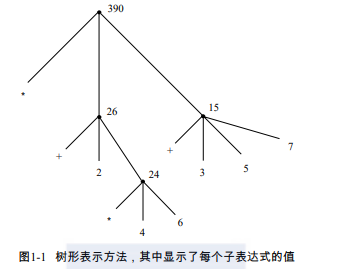### 1.1.4 复合过程

• 数和算术运算是基本的数据和过程。
• 组合式的嵌套提供了一种组织起多个操作的方法。
• 定义是一种受限的抽象手段，它为名字关联相应的值。

(define (square x) (* x x))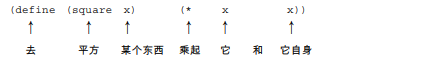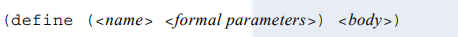(square 21)
441
(square (+ 2 5))
49
(square (square 3))
81

(+ (square x) (square y))

(define (sum-of-squares x y)
(+ (square x) (square y)))
(sum-of-squares 3 4)
25

(define (f a)
(sum-of-squares (+ a 1) (* a 2)))
(f 5)
136

### 1.1.5 过程应用的代换模型

• 将复合过程应用于实际参数，就是在将过程体中的每个形参用相应的实参取代之后，对这一过程体求值。

(f 5)

(sum-of-squares (+ a 1) (* a 2))

(sum-of-squares (+ 5 1) (* 5 2))

(+ (square 6) (square 10))

(+ (* 6 6) (* 10 10))

(+ 36 100)

136

• 代换的作用只是为了帮助我们领会过程调用中的情况，而不是对解释器实际工作方式的具体描述。通常的解释器都不采用直接操作过程的正文，用值去代换形式参数的方式去完成对过程调用的求值。在实际中，它们一般采用提供形式参数的局部环境的方式，产生“代换”的效果。我们将在第3章和第4章考察一个解释器的细节实现，在那里更完整地讨论这一问题。
• 随着本书讨论的进展，我们将给出有关解释器如何工作的一系列模型，一个比一个更精细，并最终在第5章给出一个完整的解释器和一个编译器。这里的代换模型只是这些模型中的第一个—作为形式化地考虑这种求值过程的起点。一般来说，在模拟科学研究或者工程中的现象时，我们总是从最简单的不完全的模型开始。随着更细致地检查所考虑的问题，这些简单模型也会变得越来越不合适，从而必须用进一步精化的模型取代。代换模型也不例外。特别地，在第3章中，我们将要讨论将过程用于“变化的数据”的问题，那时就会看到替换模型完全不行了，必须用更复杂的过程应用模型来代替它。

(f 5)

(sum-of-squares (+ 5 1) (* 5 2))
(+    (square (+ 5 1))      (square (* 5 2))  )
(+    (* (+ 5 1) (+ 5 1))   (* (* 5 2) (* 5 2)))

(+         (* 6 6)             (* 10 10))
(+           36                   100)
136

(* x x)

Lisp采用应用序求值，部分原因在于这样做能避免对于表达式的重复求值（例如上面的（＋5 1）和（* 5 2）的情况），从而可以提高一些效率。更重要的是，在超出了可以采用替换方式模拟的过程范围之后，正则序的处理将变得更复杂。而在另一些方面，正则序也可以成为特别有价值的工具，我们将在第3章和第4章研究它的某些内在性质16。

### 1.1.6 条件表达式和谓词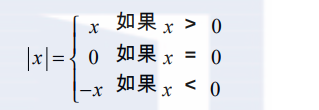(define (abs x)
(cond ((> x 0) x)
((= x 0) 0)
((< x 0) (- x))))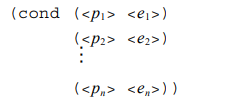(define (abs x)
(cond ((< x 0) (- x))
(else x)))

(define (abs x)
(if (< x 0)
(- x)
x))

(if <predicate> <consequent> <alternative>)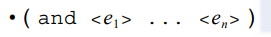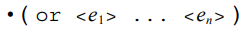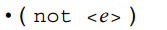(and (> x 5) (< x 10))

(define (>= x y)
(or (> x y) (= x y)))

(define (>= x y)
(not (< x y)))

10
(+ 5 3 4)
(- 9 1)
(/ 6 2)
(+ (* 2 4) (- 4 6))
(define a 3)
(define b (+ a 1))
(+ a b (* a b))
(= a b)
(if (and (> b a) (< b (* a b)))
b
a)
(cond ((= a 4) 6)
((= b 4) (+ 6 7 a))
(else 25))
(+ 2 (if (> b a) b a))
(* (cond ((> a b) a)
((< a b) b)
(else -1))
(+ a 1))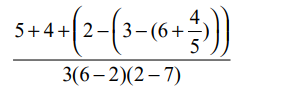(define (a-plus-abs-b a b)
((if (> b 0) + -) a b))

(define (p) (p))
(define (test x y)
(if (= x 0)
0
y))

(test 0 (p))

### 1.1.7 实例：采用牛顿法求平方根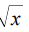＝那样的y，使得y≥0而且y^2＝x

(define (sqrt x)
(the y (and (>= y 0)
(= (square y) x))))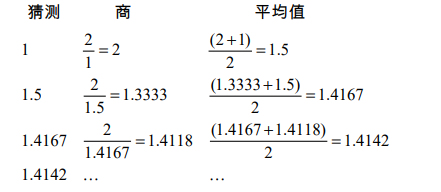(define (sqrt-iter guess x)
(if (good-enough-  guess x)
guess
(sqrt-iter (improve guess x)
x)))

(define (improve guess x)
(average guess (/ x guess)))

(define (average x y)
(/ (+ x y) 2))

(define (good-enough-  guess x)
(< (abs (- (square guess) x)) 0.001))

(define (sqrt x)
(sqrt-iter 1.0 x))

(sqrt 9)
3.00009155413138
(sqrt (+ 100 37))
11.704699917758145
(sqrt (+ (sqrt 2) (sqrt 3)))
1.7739279023207892
(square (sqrt 1000))
1000.000369924366

(define (new-if predicate then-clause else-clause)
(cond (predicate then-clause)
(else else-clause)))

Eva给Alyssa演示她的程序：

(new-if (= 2 3) 0 5)
5
(new-if (= 1 1) 0 5)
0

(define (sqrt-iter guess x)
(new-if (good-enough-  guess x)
guess
(sqrt-iter (improve guess x)
x)))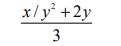### 1.1.8 过程作为黑箱抽象

sqrt是我们用一组手工定义的过程来实现一个计算过程的第一个例子。请注意，在这里sqrt-iter的定义是递归的，也就是说，这一过程的定义基于它自身。能够基于一个过程自身来定义它的想法很可能会令人感到不安，人们可能觉得它不够清晰，这种“循环”定义怎么能有意义呢？是不是完全刻画了一个能够由计算机实现的计算过程呢？在1.2节里，我们将更细致地讨论这一问题。现在首先来看看sqrt实例所显示出的其他一些要点。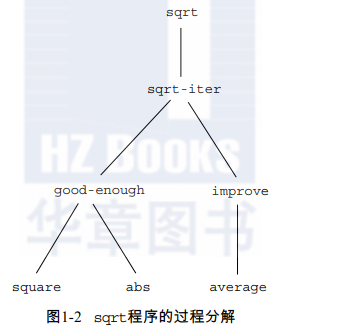(define (square x) (* x x))
(define (square x)
(exp (double (log x))))
(define (double x) (+ x x))

(define (square x) (* x x))
(define (square y) (* y y))

(define (good-enough-  guess x)
(< (abs (- (square guess) x)) 0.001))

good-enough?作者的意图就是要去确定，函数的第一个参数的平方是否位于第二个参数附近一定的误差范围内。可以看到，good-enough?的作者用名字guess表示其第一个参数，用x表示第二个参数，而送给square的实际参数就是guess。如果square的作者也用x（上面确实如此）表示参数，那么就可以明显看出，good-enough?里的x必须与square里的那个x不同。在过程square运行时，绝不应该影响good-enough?里所用的那个x的值，因为在square完成计算之后，good-enough?里可能还需要用x的值。

(define (sqrt x)
(sqrt-iter 1.0 x))
(define (sqrt-iter guess x)
(if (good-enough-  guess x)
guess
(sqrt-iter (improve guess x) x)))
(define (good-enough-  guess x)
(< (abs (- (square guess) x)) 0.001))
(define (improve guess x)
(average guess (/ x guess)))

(define (sqrt x)
(define (good-enough-  guess x)
(< (abs (- (square guess) x)) 0.001))
(define (improve guess x)
(average guess (/ x guess)))
(define (sqrt-iter guess x)
(if (good-enough-  guess x)
guess
(sqrt-iter (improve guess x) x)))
(sqrt-iter 1.0 x))

(define (sqrt x)
(define (good-enough-  guess)
(< (abs (- (square guess) x)) 0.001))
(define (improve guess)
(average guess (/ x guess)))
(define (sqrt-iter guess)
(if (good-enough-  guess)
guess
(sqrt-iter (improve guess))))
(sqrt-iter 1.0))

## 1.2 过程及其产生的计算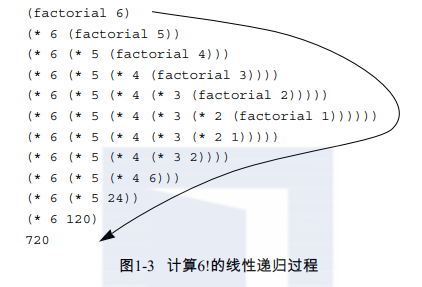### 1.2.1 线性的递归和迭代

n!＝n·(n－1)·(n－2) …3·2·1

n!＝n·[(n－1)·(n－2) …3·2·1]＝n·(n－1)!

(define (factorial n)
(if (= n 1)
1
(* n (factorial (- n 1)))))

product ← counter·product
counter ← counter + 1

(define (factorial n)
(fact-iter 1 1 n))
(define (fact-iter product counter max-count)
(if (> counter max-count)
product
(fact-iter (* counter product)
(+ counter 1)
max-count)))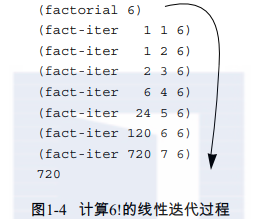(define (+ a b)
(if (= a 0)
b
(inc (+ (dec a) b))))
(define (+ a b)
(if (= a 0)
b
(+ (dec a) (inc b))))

(define (A x y)
(cond ((= y 0) 0)
((= x 0) (* 2 y))
((= y 1) 2)
(else (A (- x 1)
(A x (- y 1))))))

(A 1 10)
(A 2 4)
(A 3 3)

(define (f n) (A 0 n))
(define (g n) (A 1 n))
(define (h n) (A 2 n))
(define (k n) (* 5 n n))

### 1.2.2 树形递归

0, 1, 1, 2, 3, 5, 8, 13, 21, …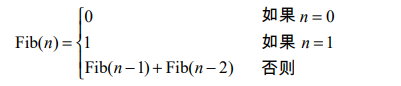(define (fib n)
(cond ((= n 0) 0)
((= n 1) 1)
(else (+ (fib (- n 1))
(fib (- n 2))))))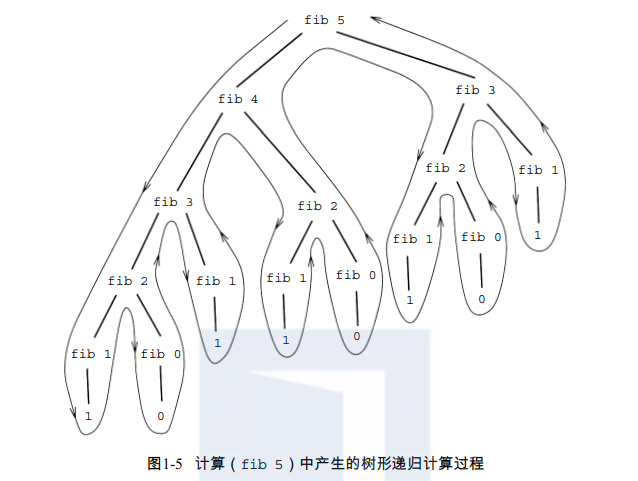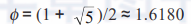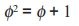a←a＋b b←a

(define (fib n)
(fib-iter 1 0 n))
(define (fib-iter a b count)
(if (= count 0)
b
(fib-iter (+ a b) a (- count 1))))

• 将现金数a换成除第一种硬币之外的所有其他硬币的不同方式数目，加上
• 将现金数a－d换成所有种类的硬币的不同方式数目，其中的d是第一种硬币的币值。

• 如果a就是0，应该算作是有1种换零钱的方式。
• 如果a小于0，应该算作是有0种换零钱的方式。
• 如果n是0，应该算作是有0种换零钱的方式。

(define (count-change amount)
(cc amount 5))
(define (cc amount kinds-of-coins)
(cond ((= amount 0) 1)
((or (< amount 0) (= kinds-of-coins 0)) 0)
(else (+ (cc amount
(- kinds-of-coins 1))
(cc (- amount
(first-denomination kinds-of-coins))
kinds-of-coins)))))
(define (first-denomination kinds-of-coins)
(cond ((= kinds-of-coins 1) 1)
((= kinds-of-coins 2) 5)
((= kinds-of-coins 3) 10)
((= kinds-of-coins 4) 25)
((= kinds-of-coins 5) 50)))

（过程first-denomination以可用的硬币种数作为输入，返回第一种硬币的币值。这里认为硬币已经从最大到最小排列好了，其实采用任何顺序都可以）。我们现在就能回答开始的问题了，下面是换1美元硬币的不同方式数目：

(count-change 100)
292

count-change产生出一个树形的递归计算过程，其中的冗余计算与前面fib的第一种实现类似（它计算出292需要一点时间）。另一方面，要想设计出一个更好的算法，使之能算出同样结果，就不那么明显了。我们将这一问题留给读者作为一个挑战。人们认识到，树形递归计算过程有可能极其低效，但常常很容易描述和理解，这就导致人们提出了一个建议，希望能利用世界上的这两个最好的东西。人们希望能设计出一种“灵巧编译器”，使之能将一个树形递归的过程翻译为一个能计算出同样结果的更有效的过程34。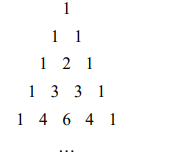### 1.2.3 增长的阶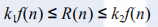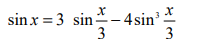(define (cube x) (* x x x))
(define (p x) (- (* 3 x) (* 4 (cube x))))
(define (sine angle)
(if (not (> (abs angle) 0.1))
angle
(p (sine (/ angle 3.0)))))

a) 在求值（sine 12.15）时，p将被使用多少次？
b) 在求值（sine a）时，由过程sine所产生的计算过程使用的空间和步数（作为a的函数）增长的阶是什么？

### 1.2.4 求幂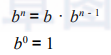(define (expt b n)
(if (= n 0)
1
(* b (expt b (- n 1)))))

(define (expt b n)
(expt-iter b n 1))
(define (expt-iter b counter product)
(if (= counter 0)
product
(expt-iter b
(- counter 1)
(* b product))))

b·(b·(b·(b·(b·(b·(b·b))))))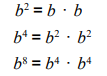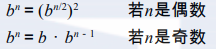(define (fast-expt b n)
(cond ((= n 0) 1)
((even? n) (square (fast-expt b (/ n 2))))
(else (* b (fast-expt b (- n 1))))))

(define (even? n)
(= (remainder n 2) 0))

(define (* a b)
(if (= b 0)
0
(+ a (* a (- b 1)))))

(define (fib n)
(fib-iter 1 0 0 1 n))
(define (fib-iter a b p q count)
(cond ((= count 0) b)
((even? count)
(fib-iter a
b
<??>      ; compute p'
<??>      ; compute q'
(/ count 2)))
(else (fib-iter (+ (* b q) (* a q) (* a p))
(+ (* b p) (* a q))
p
q
(- count 1)))))

### 1.2.5 最大公约数

GCD(a, b)＝GCD(b, r)

GCD(206, 40)＝GCD(40, 6) ＝GCD(6, 4) ＝GCD(4, 2) ＝GCD(2, 0) ＝2

(define (gcd a b)
(if (= b 0)
a
(gcd b (remainder a b))))

Lamé定理：如果欧几里得算法需要用k步计算出一对整数的GCD，那么这对数中较小的那个数必然大于或者等于第k个斐波那契数。

### 1.2.6 实例：素数检测

(define (smallest-divisor n)
(find-divisor n 2))
(define (find-divisor n test-divisor)
(cond ((> (square test-divisor) n) n)
((divides? test-divisor n) test-divisor)
(else (find-divisor n (+ test-divisor 1)))))
(define (divides? a b)
(= (remainder b a) 0))

(define (prime? n)
(= n (smallest-divisor n)))

find-divisor的结束判断基于如下事实，如果n不是素数，它必然有一个小于或者等于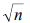的因子。这也意味着该算法只需在1和之间检查因子。由此可知，确定是否素数所需的步数将具有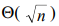的增长阶。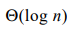的素数检查基于数论中著名的费马小定理的结果45。

（两个数称为是模n同余，如果它们除以n的余数相同。数a除以n的余数称为a取模n的余数，或简称为a取模n）。

(define (expmod base exp m)
(cond ((= exp 0) 1)
((even? exp)
(remainder (square (expmod base (/ exp 2) m))
m))
(else
(remainder (* base (expmod base (- exp 1) m))
m))))

(define (fermat-test n)
(define (try-it a)
(= (expmod a n n) a))
(try-it (+ 1 (random (- n 1)))))

(define (fast-prime? n times)
(cond ((= times 0) true)
((fermat-test n) (fast-prime? n (- times 1)))
(else false)))

(define (timed-prime-test n)
(newline)
(display n)
(start-prime-test n (runtime)))
(define (start-prime-test n start-time)
(if (prime? n)
(report-prime (- (runtime) start-time))))
(define (report-prime elapsed-time)
(display " *** ")
(display elapsed-time))

(define (expmod base exp m)
(remainder (fast-expt base exp) m))

(define (expmod base exp m)
(cond ((= exp 0) 1)
((even? exp)
(remainder (* (expmod base (/ exp 2) m)
(expmod base (/ exp 2) m))
m))
(else
(remainder (* base (expmod base (- exp 1) m))
m))))

“我看不出来这会造成什么不同，”Louis说。“我能看出，”Eva说，“采用这种方式写出该过程时，你就把一个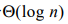的计算过程变成Θ(n) 的了。”请解释这一问题。

## 1.3 用高阶函数做抽象

(define (cube x) (* x x x))

(* 3 3 3)
(* x x x)
(* y y y)

### 1.3.1 过程作为参数

(define (sum-integers a b)
(if (> a b)
0
(+ a (sum-integers (+ a 1) b))))

(define (sum-cubes a b)
(if (> a b)
0
(+ (cube a) (sum-cubes (+ a 1) b))))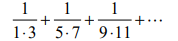(define (pi-sum a b)
(if (> a b)
0
(+ (/ 1.0 (* a (+ a 2))) (pi-sum (+ a 4) b))))

(define (<name> a b)
(if (> a b)
0
(+ (<term> a)
(<name> (<next> a) b))))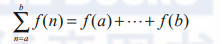(define (sum term a next b)
(if (> a b)
0
(+ (term a)
(sum term (next a) next b))))

(define (inc n) (+ n 1))
(define (sum-cubes a b)
(sum cube a inc b))

(sum-cubes 1 10)
3025

(define (identity x) x)
(define (sum-integers a b)
(sum identity a inc b))

(sum-integers 1 10)
55

(define (pi-sum a b)
(define (pi-term x)
(/ 1.0 (* x (+ x 2))))
(define (pi-next x)
(+ x 4))
(sum pi-term a pi-next b))

(* 8 (pi-sum 1 1000))
3.139592655589783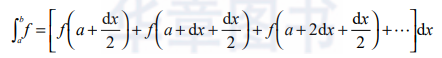(define (integral f a b dx)
(define (add-dx x) (+ x dx))
(* (sum f (+ a (/ dx 2.0)) add-dx b)
dx))
(integral cube 0 1 0.01)
.24998750000000042
(integral cube 0 1 0.001)
.249999875000001

（cube在0和1间积分的精确值是1/4。）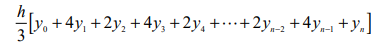(define (sum term a next b)
(define (iter a result)
(if <??>
<??>
(iter <??> <??>)))
(iter <??> <??>))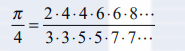b) 如果你的product过程生成的是一个递归计算过程，那么请写出一个生成迭代计算过程的过程。如果它生成一个迭代计算过程，请写一个生成递归计算过程的过程。

(accumulate combiner null-value term a next b)

accumulate取的是与sum和product一样的项和范围描述参数，再加上一个（两个参数的）combiner过程，它描述如何将当前项与前面各项的积累结果组合起来，另外还有一个null-value参数，它描述在所有的项都用完时的基本值。请写出accumulate，并说明我们能怎样基于简单地调用accumulate，定义出sum和product来。
b) 如果你的accumulate过程生成的是一个递归计算过程，那么请写出一个生成迭代计算过程的过程。如果它生成一个迭代计算过程，请写一个生成递归计算过程的过程。

a) 求出在区间a到b中所有素数之和（假定你已经写出了谓词prime?）。
b) 小于n的所有与n互素的正整数（即所有满足GCD(i, n)＝1的整数i＜n）之乘积。

### 1.3.2 用lambda构造过程

(lambda (x) (+ x 4))

(lambda (x) (/ 1.0 (* x (+ x 2))))

(define (pi-sum a b)
(sum (lambda (x) (/ 1.0 (* x (+ x 2))))
a
(lambda (x) (+ x 4))
b))

(define (integral f a b dx)
(* (sum f
(+ a (/ dx 2.0))
(lambda (x) (+ x dx))
b)
dx))

(lambda (\<formal-parameters>) \<body>)

(define (plus4 x) (+ x 4))

(define plus4 (lambda (x) (+ x 4)))

    (lambda    (x)    (+    x        4))
↑    ↑    ↑    ↑        ↑
该过程    以x为参数    它加起    x    和    4

((lambda (x y z) (+ x y (square z))) 1 2 3)
12

lambda的另一个应用是创建局部变量。在一个过程里，除了使用那些已经约束为过程参数的变量外，我们常常还需要另外一些局部变量。例如，假定我们希望计算函数：

f(x, y)＝x(1＋xy)2＋y(1－y)＋(1＋xy)(1－y)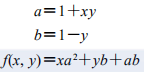(define (f x y)
(define (f-helper a b)
(+ (* x (square a))
(* y b)
(* a b)))
(f-helper (+ 1 (* x y))
(- 1 y)))

(define (f x y)
((lambda (a b)
(+ (* x (square a))
(* y b)
(* a b)))
(+ 1 (* x y))
(- 1 y)))

(define (f x y)
(let ((a (+ 1 (* x y)))
(b (- 1 y)))
(+ (* x (square a))
(* y b)
(* a b))))

let表达式的一般形式是：

(let ((<var1> <exp1>)
(<var2> <exp2>)

(<varn> <expn>))
<body>)

令 <var1> 具有值 <exp1> 而且
<var2> 具有值 <exp2> 而且

<varn> 具有值 <expn>

let表达式的第一部分是个名字-表达式对偶的表，当let被求值时，这里的每个名字将被关联于对应表达式的值。在将这些名字约束为局部变量的情况下求值let的体。这一做法正好使let表达式被解释为替代如下表达式的另一种语法形式：

((lambda (<var1> ...<varn>)
<body>)
<exp1>

<expn>)

?let使人能在尽可能接近其使用的地方建立局部变量约束。例如，如果x的值是5，下面表达式

     (+ (let ((x 3))
(+ x (* x 10)))
x)

?变量的值是在let之外计算的。在为局部变量提供值的表达式依赖于某些与局部变量同名的变量时，这一规定就起作用了。例如，如果x的值是2，表达式：

     (let ((x 3)
(y (+ x 2)))
(* x y))

     (define (f x y)
(define a (+ 1 (* x y)))
(define b (- 1 y))
(+ (* x (square a))
(* y b)
(* a b)))

(define (f g)
(g 2))

(f square)
4
(f (lambda (z) (* z (+ z 1))))
6

### 1.3.3 过程作为一般性的方法

(define (search f neg-point pos-point)
(let ((midpoint (average neg-point pos-point)))
(if (close-enough? neg-point pos-point)
midpoint
(let ((test-value (f midpoint)))
(cond ((positive? test-value)
(search f neg-point midpoint))
((negative? test-value)
(search f midpoint pos-point))
(else midpoint))))))

(define (close-enough? x y)
(< (abs (- x y)) 0.001))

search很难直接去用，因为我们可能会偶然地给了它一对点，相应的 f 值并不具有这个过程所需的正负号，这时就会得到错误的结果。让我们换一种方式，通过下面的过程去用search，这一过程检查是否某个点具有负的函数值，另一个点是正值，并根据具体情况去调用search过程。如果这一函数在两个给定点的值同号，那么就无法使用折半方法，在这种情况下过程发出错误信号。

(define (half-interval-method f a b)
(let ((a-value (f a))
(b-value (f b)))
(cond ((and (negative? a-value) (positive? b-value))
(search f a b))
((and (negative? b-value) (positive? a-value))
(search f b a))
(else
(error "Values are not of opposite sign" a b)))))

(half-interval-method sin 2.0 4.0)
3.14111328125

(half-interval-method (lambda (x) (- (* x x x) (* 2 x) 3))
1.0
2.0)
1.89306640625

f(x), f( f(x)), f( f( f(x))), . . .

(define tolerance 0.00001)
(define (fixed-point f first-guess)
(define (close-enough? v1 v2)
(< (abs (- v1 v2)) tolerance))
(define (try guess)
(let ((next (f guess)))
(if (close-enough? guess next)
next
(try next))))
(try first-guess))

(fixed-point cos 1.0)
.7390822985224023

(fixed-point (lambda (y) (+ (sin y) (cos y)))
1.0)
1.2587315962971173

(define (sqrt x)
(fixed-point (lambda (y) (/ x y))
1.0))

(define (sqrt x)
(fixed-point (lambda (y) (average y (/ x y)))
1.0))

（请注意，y＝(1/2)(y＋x/y) 是方程y＝x/y经过简单变换的结果，导出它的方式是在方程两边都加y，然后将两边都除以2。）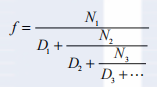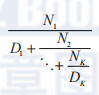(cont-frac (lambda (i) 1.0)
(lambda (i) 1.0)
k)

b) 如果你的过程产生一个递归计算过程，那么请写另一个产生迭代计算的过程。如果它产生迭代计算，请写出另一个过程，使之产生一个递归计算过程。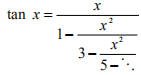### 1.3.4 过程作为返回值

(define (average-damp f)
(lambda (x) (average x (f x))))

((average-damp square) 10)
55

(define (sqrt x)
(fixed-point (average-damp (lambda (y) (/ x y)))
1.0))

(define (cube-root x)
(fixed-point (average-damp (lambda (y) (/ x (square y))))
1.0))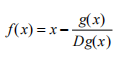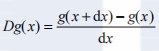(define (deriv g)
(lambda (x)
(/ (- (g (+ x dx)) (g x))
dx)))

(define dx 0.00001)

(define (cube x) (* x x x))
((deriv cube) 5)
75.00014999664018

(define (newton-transform g)
(lambda (x)
(- x (/ (g x) ((deriv g) x)))))
(define (newtons-method g guess)
(fixed-point (newton-transform g) guess))

newton-transform过程描述的就是在本节开始处的公式，基于它去定义newtons-method已经很容易了。这一过程以一个过程为参数，它计算的就是我们希望去找到零点的函数，这里还需要给出一个初始猜测。例如，为确定x的平方根，可以用初始猜测1，通过牛顿法去找函数y->y2－x的零点63。这样就给出了求平方根函数的另一种形式：

(define (sqrt x)
(newtons-method (lambda (y) (- (square y) x))
1.0))

(define (fixed-point-of-transform g transform guess)
(fixed-point (transform g) guess))

(define (sqrt x)
(fixed-point-of-transform (lambda (y) (/ x y))
average-damp
1.0))

(define (sqrt x)
(fixed-point-of-transform (lambda (y) (- (square y) x))
newton-transform
1.0))

• 可以用变量命名；
• 可以提供给过程作为参数；
• 可以由过程作为结果返回；
• 可以包含在数据结构中65。

Lisp不像其他程序设计语言，它给了过程完全的第一级状态。这就给有效实现提出了挑战，但由此所获得的描述能力却是极其惊人的66。

(newtons-method (cubic a b c) 1)

(((double (double double)) inc) 5)

((compose square inc) 6)
49

((repeated square 2) 5)
6251162 02101 0《Web前端工程师修炼之道（原书第4版）》——我需要购买些什么
1292 0《Web前端工程师修炼之道（原书第4版）》——没有足够的IP地址了
841 0《C++面向对象高效编程（第2版）》——3.9 类可以包含什么
1301 0《面向对象的思考过程（原书第4版）》一3.8　引用
989 0《面向对象的思考过程（原书第4版）》一2.5　引用
987 0Spark修炼之道系列教程预告

5955 0+关注
1170

2

+ 订阅